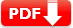Due attention is given to your level. It will guide you through the basic concepts and skills with readings activities, questions, and illustrations to support the textbook. Each unit begins with the unit outcomes and is followed by an introduction (overview).

## Ethiopian Grade 8 Physics TextBook

 Country Ethiopia Publisher MoE, Ethiopia Class Grade 8 Subject Physics Type Textbook Purpose Student Text Book Date of Uploaded June 15, 2020 Total Units 8 TextBook Version 1.0

### UNIT 1: PHYSICS AND MEASUREMENT

In the lower grades, you learned about science in general. For example, environmental science and integrated science. In this and next grades you will learn about physics, chemistry, and biology separately. In this unit you will learn what physics is and about measurement.

• 1.1 Definition of Physics
• 1.2 Standardization and Measurement
• 1.3 Measuring Length, Mass, and Time
 Download Unit 1 in PDF### UNIT 2: MOTION

In this unit, you will be introduced to the basic concepts and relationships in motion. Motion is one of the key topics in physics. Everything in the universe moves. We use some basic concepts when we express motion. These concepts are distance, displacement, speed, velocity and
acceleration.

• 2.1 Definition of Motion
• 2.2 Motion Along a Straight Line
• 2.3 Qualitative Exploration of Constant Velocity and Accelerated Motion
 Download Unit 2 in PDF### UNIT 3: FORCE AND NEWTON’S LAWS OF MOTION

In unit two of this book, you learned important concepts that describe motion. The concepts such as speed, velocity, and acceleration are used for describing various types of motion around you.

• 3.1 Force
• 3.2 Newton’s laws of motion
• 3.3 Frictional Force
 Download Unit 3 in PDF### UNI 4. WORK, ENERGY, AND POWER

In the last three units, you learned some properties of physical quantities, measurements of physical quantities, their SI units, the motion of bodies, force, and the relationship between force and motion. In this unit, you will learn the concepts of work, energy, power, and the relationship among them. What is work? How do you define energy?

• 4.1 Work
• 4.2 Energy
• 4.3 Transformation and conservation of energy
• 4.4 Power
 Download Unit 4 in PDF### UNIT 5. SIMPLE MACHINES

Group the following devices as machines or not machines: knife, scissors, screw, computer, bottle opener, axel, typewriter, electric motor, lever, pulley, and wedge. To understand the purposes of machines, you need to revise the concepts of force, work, weight, ratio, and percentage, because you often use them in this chapter.

• 5.1 Definition of machines.
• 5.2 Mechanical Advantage, Velocity Ratio and The efficiency of Machines
• 5.3 Types of Simple Machines
 Download Unit 5 in PDF### UNIT 6.TEMPERATURE AND HEAT

So far, you have studied three basic physical quantities mass, length, and time. In this unit, you will learn the fourth basic physical quantity called temperature. This unit introduces the concept of temperature and discusses the differences between temperature and heat. The unit also presents temperature measuring instruments (scales), conversion of temperature scales, sources of heat, and effects of heating.

• 6.1 Definition of Temperature
• 6.2 Measuring temperature
• 6.3 Temperature Scales
• 6.4 Conversion of Temperature Scales
• 6.5 Sources of Heat
• 6.6 Effects of Heating
 Download Unit 6 in PDF### UNIT 7. SOUND

One of the most commonly observed phenomena in nature is sound. You hear different sounds throughout the day. The sound of cars, the barking of dogs, friends chatting, a teacher talking, and music are some examples of sound. All the above-mentioned sounds stimulate your ears and make you recognize the sources of sound and the messages sent through sound.

• 7.1 Definition of Sound
• 7.2 Production and Transmission of Sound
• 7.3 Speed of Sound in Different Media
• 7.4 Reflection of Sound (Echo)
• 7.5 Application of Echo Sounding
 Download Unit 7 in PDF### UNIT 8. ELECTRICITY AND MAGNETISM

This unit is made up of two main topics called electricity and magnetism. It treats magnetism first and then electricity. Magnetism deals with the properties of a magnet while electricity deals with the properties of electrons at rest and in motion. The relationship between magnetism and electricity will be treated in grade 8.

PART-1 MAGNETISM
8.1 Magnets
8.2 Mapping Magnetic Lines of Force
8.3 Uses of Magnets

PART-2 ELECTRICITY
8.4 Electrostatics
8.5 Methods of Charging a Body
8.6 Law of Electrostatics
8.7 Electric Current and Potential Difference
8.8 Electric Circuit.

 Download Unit 7 in PDF